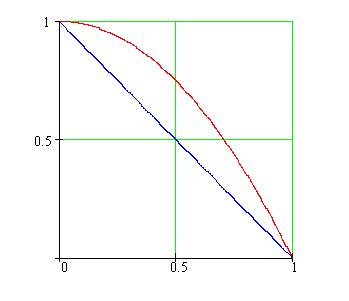Calculus on Demand at Dartmouth College Lecture 20 | Index | Lecture 22 Lecture 21## Resources

Math 3 Course Syllabus
Practice Exams

# Contents

In this lecture we introduce the main ideas of integral calculus; and give an overview of the theme that unifies lectures 21-26, modeling accumulations.

### Quick Question

What is the approximate area under the parabola and above the interval [0,1] that comes from using the triangle instead?### Outline

Outline for Modeling Accumulations

### Textbook

Modeling Accumulations